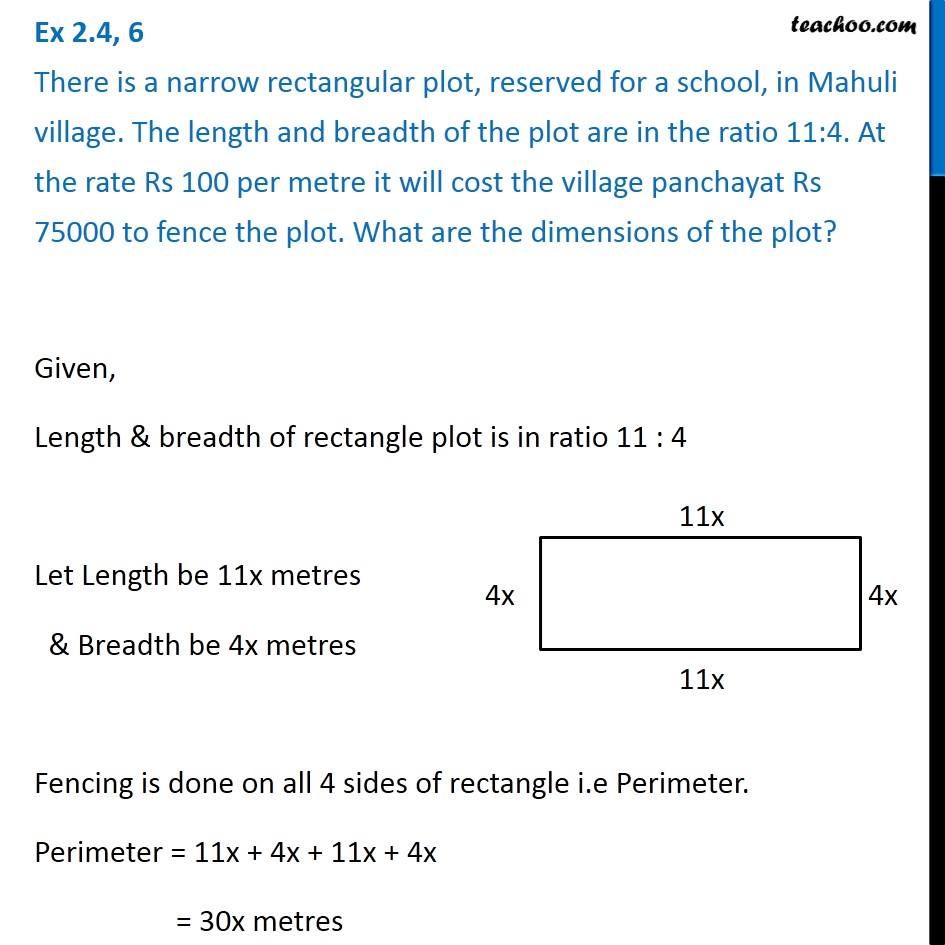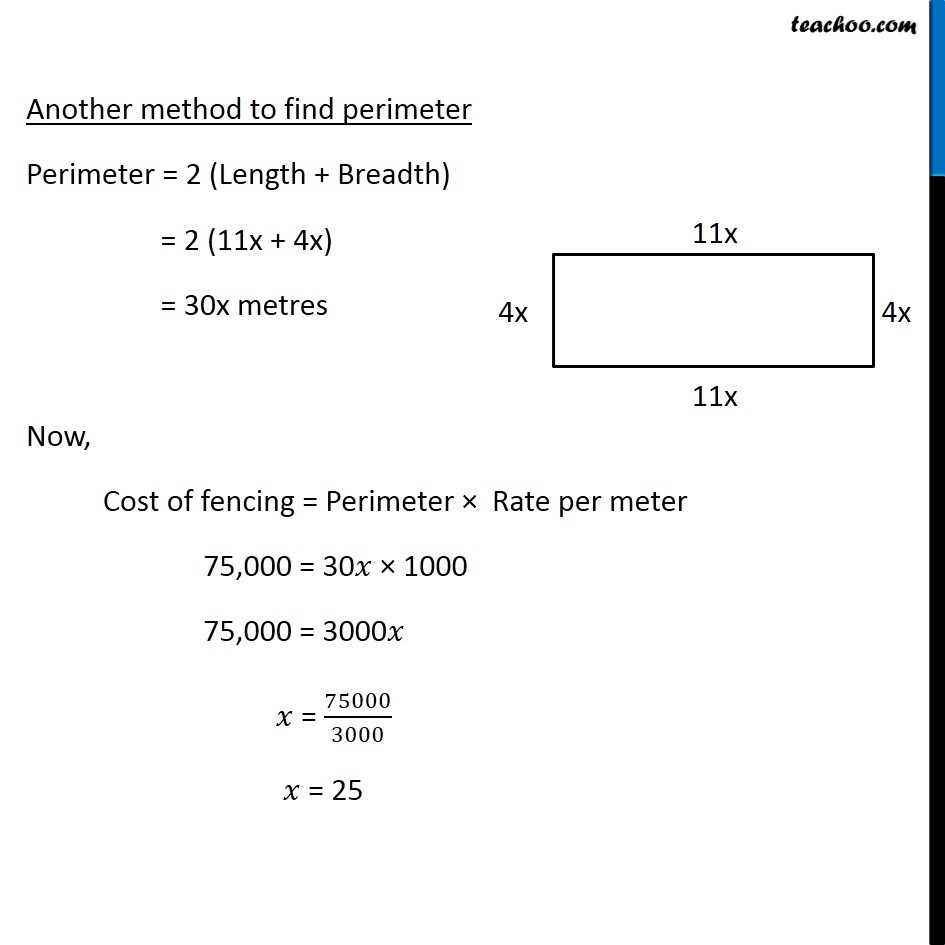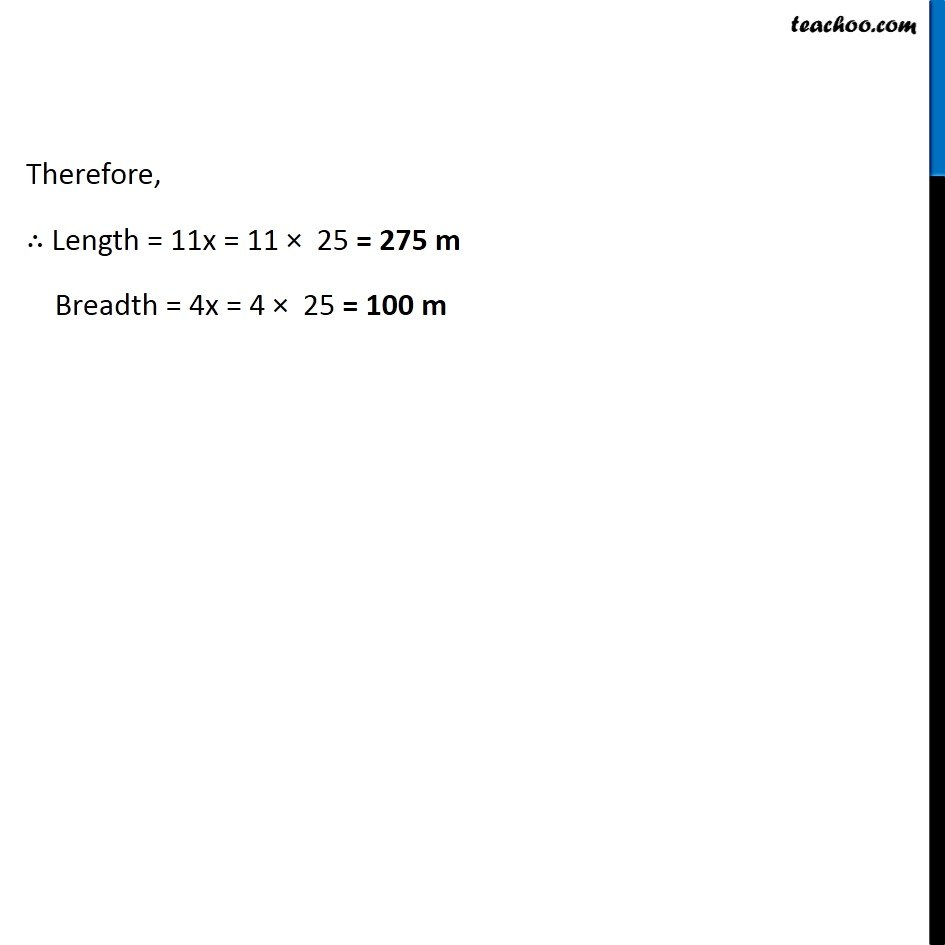Solving difficult equations

Chapter 2 Class 8 Linear Equations in One Variable
Serial order wiseLearn in your speed, with individual attention - Teachoo Maths 1-on-1 Class

### Transcript

Question 6 There is a narrow rectangular plot, reserved for a school, in Mahuli village. The length and breadth of the plot are in the ratio 11:4. At the rate Rs 100 per metre it will cost the village panchayat Rs 75000 to fence the plot. What are the dimensions of the plot?Given, Length & breadth of rectangle plot is in ratio 11 : 4 Let Length be 11x metres & Breadth be 4x metres Fencing is done on all 4 sides of rectangle i.e Perimeter. Perimeter = 11x + 4x + 11x + 4x = 30x metres Another method to find perimeter Perimeter = 2 (Length + Breadth) = 2 (11x + 4x) = 30x metres Now, Cost of fencing = Perimeter Rate per meter 75,000 = 30 1000 75,000 = 3000 = 75000/3000 = 25 Therefore, Length = 11x = 11 25 = 275 m Breadth = 4x = 4 25 = 100 m